# Suppose you want to invest \$ 1 million and you have two assets to invest in: Risk free asset with...

Suppose you want to invest \$ 1 million and you have two assets to invest in: Risk free asset with return of 12% per year and a risky asset with expected return of 30% and standard deviation of 40%. If you want a portfolio with standard deviation of 30% how much do you invest in each of the assets?

Let the weight allocated to Risk free asset be w1 and risky asset be w2 = 1 - w1

Standard deviation of risk free asset = σ1 = 0

Standard deviation of risky asset = σ2 = 0.40

Covariance between risky asset and risk free asset = Cov(1, 2) = 0 (since the covariance/correlation with risk free security is always zero)

Standard deviation of the portfolio = P = 0.30

P = [ w12σ12 + w22σ22 + 2w1w2Cov(1, 2) ]1/2 = [ w1202 + (1-w1)20.402 + 2w1(1 - w1)*0 ]1/2 = 0.30

=> (1-w1)*0.40 = 0.30

=> w1 = 1 - 0.30/0.40 = 0.25 or 25%

=> w2 = 1 - w1 = 1 - 0.25 = 0.75 or 75%

Hence, Investment in risk free asset = 0.25*1000000 = \$250000

Investment in risky asset = 0.74*1000000 = \$750000

##### Add Answer of: Suppose you want to invest \$ 1 million and you have two assets to invest in: Risk free asset with...
Similar Homework Help Questions
• ### Suppose that you have \$1 million and the following two opportunities from which to construct a...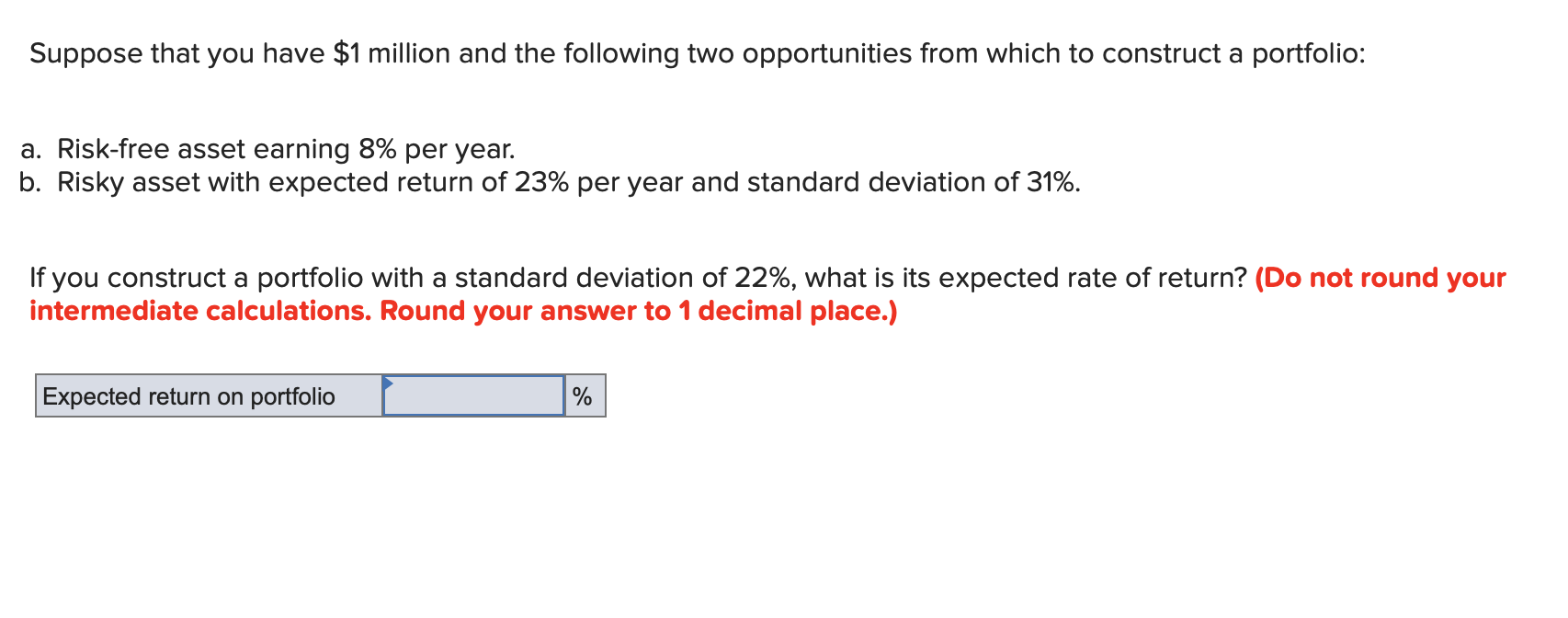Suppose that you have \$1 million and the following two opportunities from which to construct a portfolio: a. Risk-free asset earning 8% per year. b. Risky asset with expected return of 23% per year and standard deviation of 31%. If you construct a portfolio with a standard deviation of 22%, what is its expected rate of return? (Do not round your intermediate calculations. Round your answer to 1 decimal place.) Expected return on portfolio

• ### Suppose that you have \$1 million and the following two opportunities from which to construct a...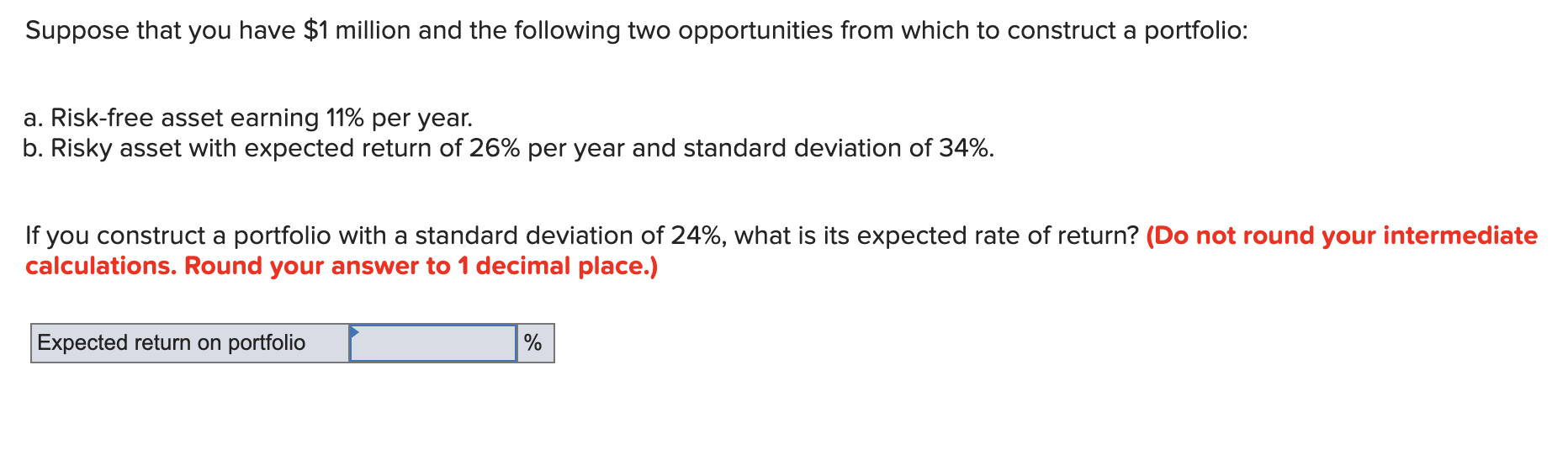Suppose that you have \$1 million and the following two opportunities from which to construct a portfolio: a. Risk-free asset earning 11% per year. b. Risky asset with expected return of 26% per year and standard deviation of 34%. If you construct a portfolio with a standard deviation of 24%, what is its expected rate of return? (Do not round your intermediate calculations. Round your answer to 1 decimal place.) Expected return on portfolio I

• ### Problem 7-16 Suppose that you have \$1 million and the following two opportunities from which to...

Problem 7-16 Suppose that you have \$1 million and the following two opportunities from which to construct a portfolio: Risk-free asset earning 11% per year. Risky asset with expected return of 26% per year and standard deviation of 34%. If you construct a portfolio with a standard deviation of 24%, what is its expected rate of return? (Do not round your intermediate calculations. Round your answer to 1 decimal place.)

• ### Question 4 (20 marks) (a) You need to invest \$10M in two assets: a risk-free asset...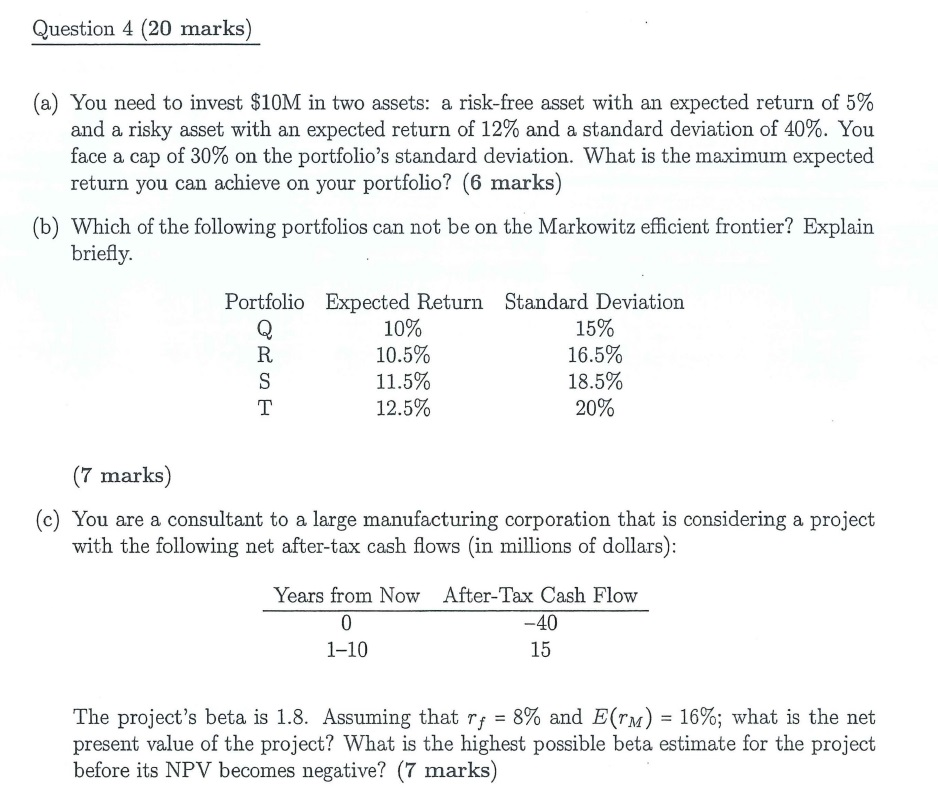Question 4 (20 marks) (a) You need to invest \$10M in two assets: a risk-free asset with an expected return of 5% and a risky asset with an expected return of 12% and a standard deviation of 40%. You face a cap of 30% on the portfolio's standard deviation. What is the maximum expected return you can achieve on your portfolio? (6 marks) (b) Which of the following portfolios can not be on the Markowitz efficient frontier? Explain briefly. Portfolio...

• ### You invest \$100 in a complete portfolio. The complete portfolio is composed of a risky asset...

You invest \$100 in a complete portfolio. The complete portfolio is composed of a risky asset with an expected rate of return of 12% and a standard deviation of 10% and a treasury bill with a rate of return of 5%. __________ of your complete portfolio should be invested in the risk-free asset if you want your complete portfolio to have a standard deviation of 9%.

• ### Question 1: Suppose there are two risky assets, A and B. You collect the following data...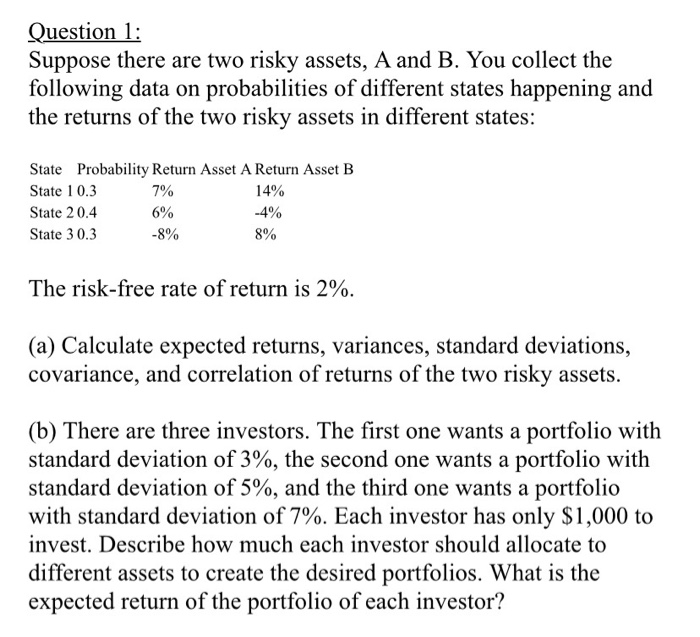Question 1: Suppose there are two risky assets, A and B. You collect the following data on probabilities of different states happening and the returns of the two risky assets in different states: State Probability Return Asset A Return Asset B State 10.3 7% 14% State 20.4 6% -4% State 30.3 -8% 8% The risk-free rate of return is 2%. (a) Calculate expected returns, variances, standard deviations, covariance, and correlation of returns of the two risky assets. (b) There are...

• ### The universe of available securities includes two risky stocks A and B, and a risk-free asset....

The universe of available securities includes two risky stocks A and B, and a risk-free asset. The data for the universe are as follows: Assets Expected Return Standard Deviation Stock A 6% 25% Stock B 12% 42% Risk free 5% 0 The correlation coefficient between A and B is -0.2. The investor maximizes a utility function U=E(r)−σ2 (i.e. she has a coefficient of risk aversion equal to 2). Assume that to maximize his utility when there is no available risk-free...

• ### 12 You want to create a portfolio equally as risky as the market, and you have \$1,000,000 to invest. You must invest al...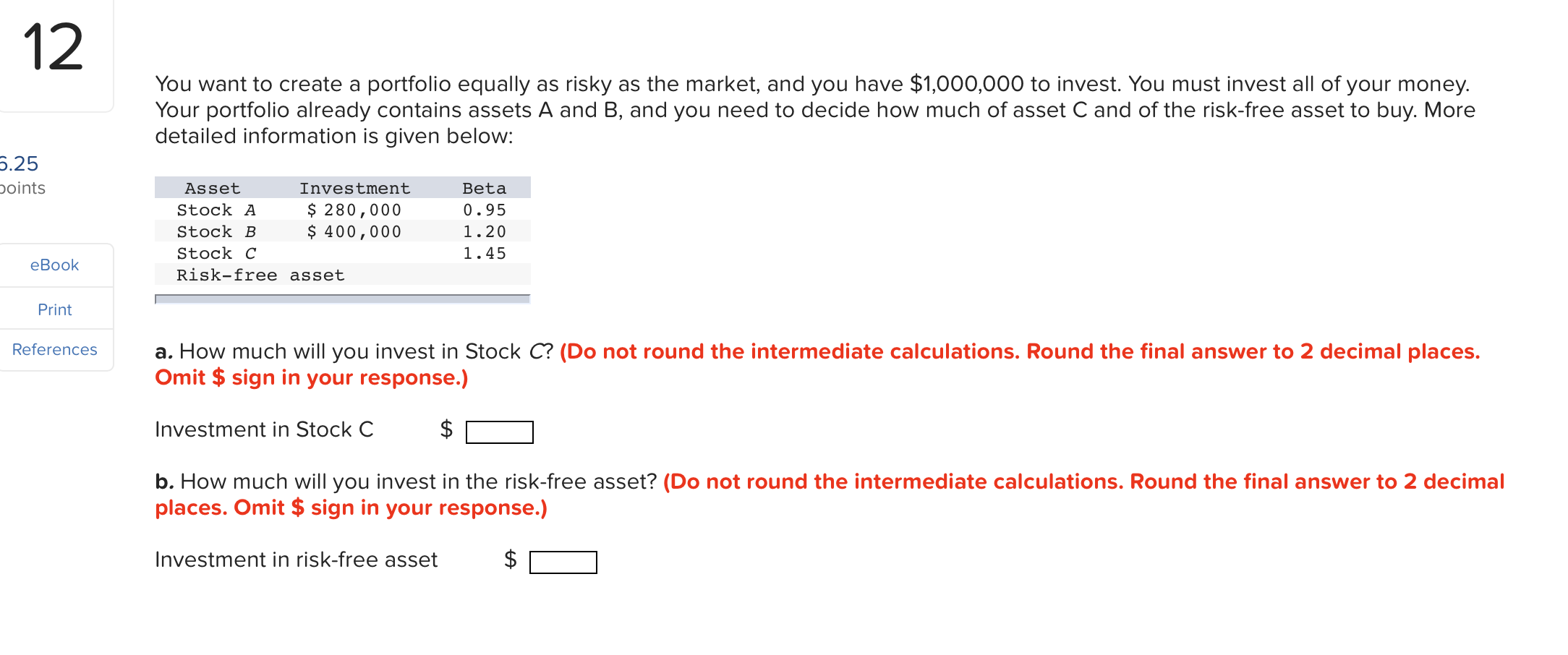12 You want to create a portfolio equally as risky as the market, and you have \$1,000,000 to invest. You must invest all of your money. Your portfolio already contains assets A and B, and you need to decide how much of asset C and of the risk-free asset to buy. More detailed information is given below: 5.25 points Asset Investment Stock A \$ 280,000 Stock B \$ 400,000 Stock C Risk-free asset Beta 0.95 1.20 1.45 eBook Print References...

• ### You are presented with information on expected returns and standard deviations for 2 assets and a...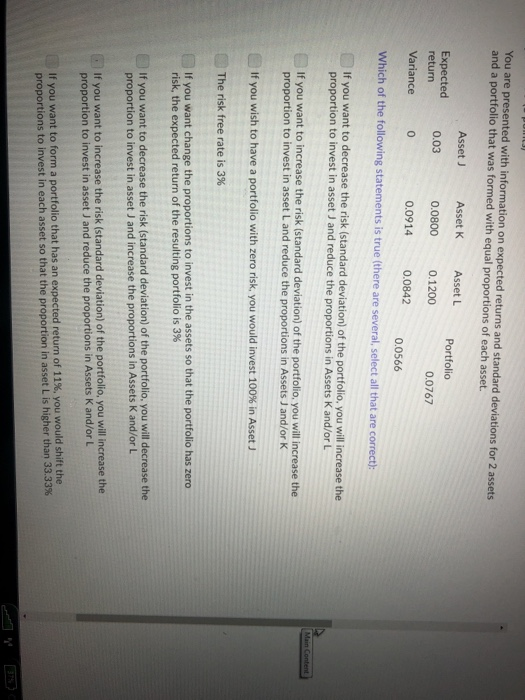You are presented with information on expected returns and standard deviations for 2 assets and a portfolio that was formed with equal proportions of each asset. Asset J Asset K 00800 0.1200 0.0914 Asset L Portfolio Expected return 0.03 0.0767 Variance 0.0842 0.0566 Which of the following statements is true (there are several, select all that are correct): If you want to decrease the risk (standard deviation) of the portfolio, you will increase the proportion to invest in asset J...

• ### 2. (Understanding optimal portfolio choice) Consider two risky assets, the expected return of asset one is...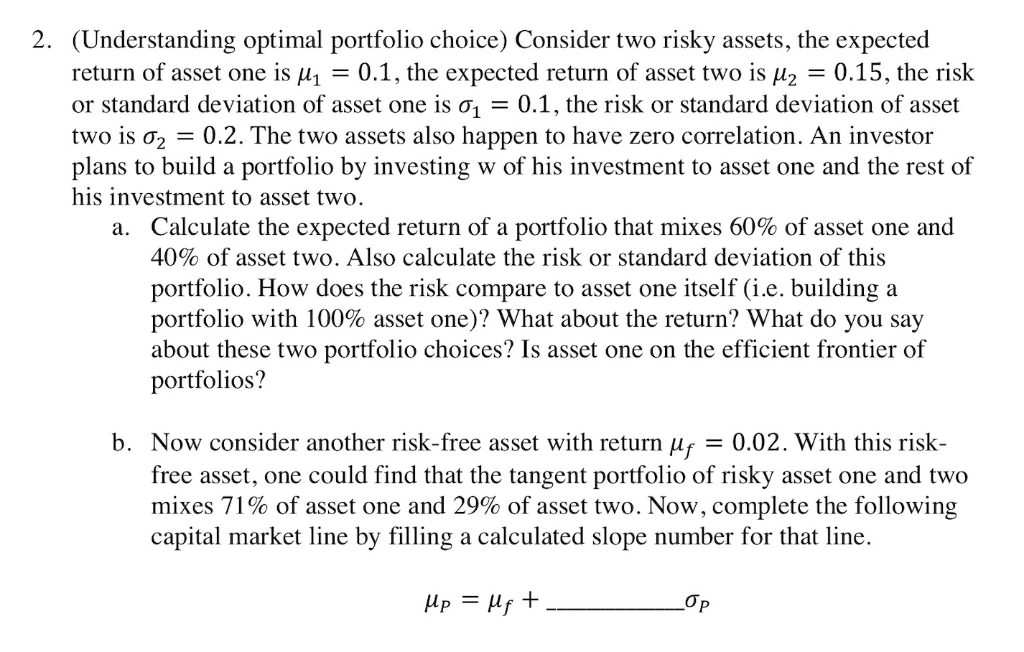2. (Understanding optimal portfolio choice) Consider two risky assets, the expected return of asset one is μ-0.1, the expected return of asset two is μ2-0.15, the risk or standard deviation of asset one is σ1-0.1, the risk or standard deviation of asset two is σ2-02. The two assets also happen to have zero correlation. An investor plans to build a portfolio by investing w of his investment to asset one and the rest of his investment to asset two. Calculate...

Need Online Homework Help?